# Mean State

Period Mean (original grids) [Watt m-2]
Model Period Mean (intersection) [Watt m-2]
Model Period Mean (complement) [Watt m-2]
Benchmark Period Mean (intersection) [Watt m-2]
Benchmark Period Mean (complement) [Watt m-2]
Bias [Watt m-2]
RMSE [Watt m-2]
Phase Shift [months]
Bias Score 
RMSE Score 
Seasonal Cycle Score 
Spatial Distribution Score 
Interannual Variability Score 
Overall Score 
Benchmark [-] 38.3
CLM4 [-] 34.6 34.4 39.6 15.5 -4.65 11.3 1.66 0.324 0.368 0.736 0.958 0.521 0.546
CLM4.5 [-] 34.2 33.9 39.6 15.5 -5.00 11.6 1.73 0.308 0.363 0.722 0.959 0.521 0.539
CLM5 [-] 33.9 33.6 39.6 15.5 -5.32 11.4 1.57 0.312 0.367 0.754 0.980 0.532 0.552
Period Mean (original grids) [Watt m-2]
Model Period Mean (intersection) [Watt m-2]
Model Period Mean (complement) [Watt m-2]
Benchmark Period Mean (intersection) [Watt m-2]
Benchmark Period Mean (complement) [Watt m-2]
Bias [Watt m-2]
RMSE [Watt m-2]
Phase Shift [months]
Bias Score 
RMSE Score 
Seasonal Cycle Score 
Spatial Distribution Score 
Interannual Variability Score 
Overall Score 
Benchmark [-] 52.3
CLM4 [-] 47.2 47.6 52.8 14.1 -5.33 13.1 1.28 0.440 0.370 0.832 0.989 0.562 0.594
CLM4.5 [-] 46.2 46.7 52.8 14.1 -6.19 13.5 1.19 0.414 0.372 0.848 0.989 0.557 0.592
CLM5 [-] 45.3 45.8 52.8 14.1 -7.07 13.8 1.27 0.396 0.379 0.845 0.990 0.530 0.587
Period Mean (original grids) [Watt m-2]
Model Period Mean (intersection) [Watt m-2]
Model Period Mean (complement) [Watt m-2]
Benchmark Period Mean (intersection) [Watt m-2]
Benchmark Period Mean (complement) [Watt m-2]
Bias [Watt m-2]
RMSE [Watt m-2]
Phase Shift [months]
Bias Score 
RMSE Score 
Seasonal Cycle Score 
Spatial Distribution Score 
Interannual Variability Score 
Overall Score 
Benchmark [-] 39.3
CLM4 [-] 37.3 37.4 40.4 16.6 -2.94 12.0 1.96 0.424 0.374 0.693 0.917 0.594 0.562
CLM4.5 [-] 36.8 36.8 40.4 16.6 -3.44 12.4 1.95 0.402 0.366 0.693 0.928 0.594 0.558
CLM5 [-] 36.6 36.6 40.4 16.6 -3.70 12.2 1.81 0.399 0.383 0.720 0.910 0.579 0.562
Period Mean (original grids) [Watt m-2]
Model Period Mean (intersection) [Watt m-2]
Model Period Mean (complement) [Watt m-2]
Benchmark Period Mean (intersection) [Watt m-2]
Benchmark Period Mean (complement) [Watt m-2]
Bias [Watt m-2]
RMSE [Watt m-2]
Phase Shift [months]
Bias Score 
RMSE Score 
Seasonal Cycle Score 
Spatial Distribution Score 
Interannual Variability Score 
Overall Score 
Benchmark [-] 31.6
CLM4 [-] 40.8 40.7 31.5 32.6 9.34 24.1 0.258 0.700 0.508 0.982 0.960 0.801 0.743
CLM4.5 [-] 35.8 35.7 31.5 32.6 5.05 19.2 0.264 0.789 0.574 0.982 0.973 0.752 0.774
CLM5 [-] 34.3 34.2 31.5 32.6 3.60 18.0 0.327 0.799 0.589 0.978 0.972 0.759 0.781
Period Mean (original grids) [Watt m-2]
Model Period Mean (intersection) [Watt m-2]
Model Period Mean (complement) [Watt m-2]
Benchmark Period Mean (intersection) [Watt m-2]
Benchmark Period Mean (complement) [Watt m-2]
Bias [Watt m-2]
RMSE [Watt m-2]
Phase Shift [months]
Bias Score 
RMSE Score 
Seasonal Cycle Score 
Spatial Distribution Score 
Interannual Variability Score 
Overall Score 
Benchmark [-] 23.5
CLM4 [-] 27.7 27.7 25.9 15.5 2.39 11.1 1.47 0.358 0.354 0.786 0.255 0.580 0.448
CLM4.5 [-] 27.5 27.5 25.9 15.5 2.24 11.1 1.47 0.351 0.356 0.786 0.278 0.574 0.450
CLM5 [-] 27.8 27.7 25.9 15.5 2.49 11.1 1.43 0.363 0.364 0.791 0.231 0.552 0.444
Period Mean (original grids) [Watt m-2]
Model Period Mean (intersection) [Watt m-2]
Model Period Mean (complement) [Watt m-2]
Benchmark Period Mean (intersection) [Watt m-2]
Benchmark Period Mean (complement) [Watt m-2]
Bias [Watt m-2]
RMSE [Watt m-2]
Phase Shift [months]
Bias Score 
RMSE Score 
Seasonal Cycle Score 
Spatial Distribution Score 
Interannual Variability Score 
Overall Score 
Benchmark [-] 21.4
CLM4 [-] 23.8 23.7 21.5 17.6 2.22 11.2 0.647 0.761 0.501 0.925 0.856 0.729 0.712
CLM4.5 [-] 26.0 25.9 21.5 17.6 4.39 13.4 0.534 0.695 0.462 0.938 0.728 0.718 0.667
CLM5 [-] 25.4 25.3 21.5 17.6 3.82 12.6 0.569 0.726 0.468 0.931 0.818 0.743 0.692
Period Mean (original grids) [Watt m-2]
Model Period Mean (intersection) [Watt m-2]
Model Period Mean (complement) [Watt m-2]
Benchmark Period Mean (intersection) [Watt m-2]
Benchmark Period Mean (complement) [Watt m-2]
Bias [Watt m-2]
RMSE [Watt m-2]
Phase Shift [months]
Bias Score 
RMSE Score 
Seasonal Cycle Score 
Spatial Distribution Score 
Interannual Variability Score 
Overall Score 
Benchmark [-] 30.0
CLM4 [-] 36.2 36.1 30.5 14.9 5.01 11.9 0.933 0.642 0.475 0.881 0.907 0.657 0.673
CLM4.5 [-] 37.0 37.0 30.5 14.9 5.79 12.3 0.946 0.610 0.469 0.876 0.912 0.663 0.666
CLM5 [-] 35.3 35.3 30.5 14.9 4.16 11.4 0.960 0.671 0.469 0.872 0.973 0.670 0.687
Period Mean (original grids) [Watt m-2]
Model Period Mean (intersection) [Watt m-2]
Model Period Mean (complement) [Watt m-2]
Benchmark Period Mean (intersection) [Watt m-2]
Benchmark Period Mean (complement) [Watt m-2]
Bias [Watt m-2]
RMSE [Watt m-2]
Phase Shift [months]
Bias Score 
RMSE Score 
Seasonal Cycle Score 
Spatial Distribution Score 
Interannual Variability Score 
Overall Score 
Benchmark [-] 29.5
CLM4 [-] 34.3 34.2 30.6 14.2 3.13 12.3 0.998 0.581 0.378 0.886 0.965 0.566 0.626
CLM4.5 [-] 33.9 33.8 30.6 14.2 2.71 12.2 0.978 0.567 0.381 0.885 0.972 0.545 0.622
CLM5 [-] 33.5 33.4 30.6 14.2 2.29 11.6 0.992 0.588 0.397 0.884 0.977 0.514 0.626
Period Mean (original grids) [Watt m-2]
Model Period Mean (intersection) [Watt m-2]
Model Period Mean (complement) [Watt m-2]
Benchmark Period Mean (intersection) [Watt m-2]
Benchmark Period Mean (complement) [Watt m-2]
Bias [Watt m-2]
RMSE [Watt m-2]
Phase Shift [months]
Bias Score 
RMSE Score 
Seasonal Cycle Score 
Spatial Distribution Score 
Interannual Variability Score 
Overall Score 
Benchmark [-] 33.0
CLM4 [-] 37.8 37.6 32.8 37.1 4.93 21.2 0.212 0.791 0.551 0.986 0.824 0.788 0.749
CLM4.5 [-] 35.1 34.9 32.8 37.1 2.57 19.1 0.244 0.811 0.585 0.984 0.904 0.741 0.768
CLM5 [-] 32.3 32.2 32.8 37.1 -0.0780 17.2 0.288 0.824 0.610 0.981 0.848 0.728 0.767
Period Mean (original grids) [Watt m-2]
Model Period Mean (intersection) [Watt m-2]
Model Period Mean (complement) [Watt m-2]
Benchmark Period Mean (intersection) [Watt m-2]
Benchmark Period Mean (complement) [Watt m-2]
Bias [Watt m-2]
RMSE [Watt m-2]
Phase Shift [months]
Bias Score 
RMSE Score 
Seasonal Cycle Score 
Spatial Distribution Score 
Interannual Variability Score 
Overall Score 
Benchmark [-] 35.1
CLM4 [-] 41.0 41.0 36.7 14.1 3.17 10.4 0.491 0.605 0.536 0.958 0.920 0.608 0.694
CLM4.5 [-] 41.0 41.0 36.7 14.1 3.20 10.4 0.466 0.610 0.536 0.959 0.936 0.603 0.697
CLM5 [-] 39.7 39.8 36.7 14.1 2.08 10.6 0.510 0.585 0.527 0.956 0.885 0.646 0.688
Period Mean (original grids) [Watt m-2]
Model Period Mean (intersection) [Watt m-2]
Model Period Mean (complement) [Watt m-2]
Benchmark Period Mean (intersection) [Watt m-2]
Benchmark Period Mean (complement) [Watt m-2]
Bias [Watt m-2]
RMSE [Watt m-2]
Phase Shift [months]
Bias Score 
RMSE Score 
Seasonal Cycle Score 
Spatial Distribution Score 
Interannual Variability Score 
Overall Score 
Benchmark [-] 19.8
CLM4 [-] 23.5 23.5 20.7 10.0 2.65 11.6 0.885 0.684 0.507 0.905 0.971 0.667 0.707
CLM4.5 [-] 22.4 22.4 20.7 10.0 1.68 10.4 1.14 0.714 0.518 0.858 0.898 0.696 0.700
CLM5 [-] 22.5 22.5 20.7 10.0 1.77 10.1 1.13 0.714 0.527 0.867 0.870 0.680 0.697
Period Mean (original grids) [Watt m-2]
Model Period Mean (intersection) [Watt m-2]
Model Period Mean (complement) [Watt m-2]
Benchmark Period Mean (intersection) [Watt m-2]
Benchmark Period Mean (complement) [Watt m-2]
Bias [Watt m-2]
RMSE [Watt m-2]
Phase Shift [months]
Bias Score 
RMSE Score 
Seasonal Cycle Score 
Spatial Distribution Score 
Interannual Variability Score 
Overall Score 
Benchmark [-] 23.6
CLM4 [-] 46.3 46.3 43.1 14.1 2.21 15.1 0.855 0.619 0.485 0.888 0.872 0.606 0.659
CLM4.5 [-] 45.6 45.6 43.1 14.1 1.62 14.6 0.863 0.612 0.488 0.885 0.853 0.603 0.655
CLM5 [-] 45.0 45.0 43.1 14.1 1.14 14.4 0.858 0.624 0.492 0.887 0.876 0.604 0.663
Period Mean (original grids) [Watt m-2]
Model Period Mean (intersection) [Watt m-2]
Model Period Mean (complement) [Watt m-2]
Benchmark Period Mean (intersection) [Watt m-2]
Benchmark Period Mean (complement) [Watt m-2]
Bias [Watt m-2]
RMSE [Watt m-2]
Phase Shift [months]
Bias Score 
RMSE Score 
Seasonal Cycle Score 
Spatial Distribution Score 
Interannual Variability Score 
Overall Score 
Benchmark [-] 64.3
CLM4 [-] 80.2 80.0 66.2 16.5 12.1 19.4 0.472 0.543 0.580 0.956 0.986 0.515 0.693
CLM4.5 [-] 79.8 79.6 66.2 16.5 11.6 19.1 0.477 0.547 0.582 0.955 0.986 0.516 0.695
CLM5 [-] 78.4 78.2 66.2 16.5 10.2 18.6 0.552 0.562 0.573 0.948 0.985 0.543 0.697
Period Mean (original grids) [Watt m-2]
Model Period Mean (intersection) [Watt m-2]
Model Period Mean (complement) [Watt m-2]
Benchmark Period Mean (intersection) [Watt m-2]
Benchmark Period Mean (complement) [Watt m-2]
Bias [Watt m-2]
RMSE [Watt m-2]
Phase Shift [months]
Bias Score 
RMSE Score 
Seasonal Cycle Score 
Spatial Distribution Score 
Interannual Variability Score 
Overall Score 
Benchmark [-] 34.3
CLM4 [-] 40.6 40.6 34.2 35.0 7.15 21.0 0.196 0.749 0.572 0.985 0.956 0.810 0.774
CLM4.5 [-] 37.6 37.5 34.2 35.0 4.54 18.1 0.214 0.789 0.610 0.983 0.963 0.765 0.787
CLM5 [-] 37.5 37.5 34.2 35.0 4.47 18.3 0.207 0.791 0.608 0.984 0.976 0.766 0.789
Period Mean (original grids) [Watt m-2]
Model Period Mean (intersection) [Watt m-2]
Model Period Mean (complement) [Watt m-2]
Benchmark Period Mean (intersection) [Watt m-2]
Benchmark Period Mean (complement) [Watt m-2]
Bias [Watt m-2]
RMSE [Watt m-2]
Phase Shift [months]
Bias Score 
RMSE Score 
Seasonal Cycle Score 
Spatial Distribution Score 
Interannual Variability Score 
Overall Score 
Benchmark [-] 24.8
CLM4 [-] 34.1 34.1 27.0 14.3 6.50 11.1 0.817 0.502 0.428 0.916 0.947 0.607 0.638
CLM4.5 [-] 34.3 34.3 27.0 14.3 6.96 11.3 0.852 0.516 0.424 0.911 0.909 0.612 0.632
CLM5 [-] 33.8 33.8 27.0 14.3 6.58 11.1 0.872 0.518 0.438 0.909 0.852 0.586 0.624
Period Mean (original grids) [Watt m-2]
Model Period Mean (intersection) [Watt m-2]
Model Period Mean (complement) [Watt m-2]
Benchmark Period Mean (intersection) [Watt m-2]
Benchmark Period Mean (complement) [Watt m-2]
Bias [Watt m-2]
RMSE [Watt m-2]
Phase Shift [months]
Bias Score 
RMSE Score 
Seasonal Cycle Score 
Spatial Distribution Score 
Interannual Variability Score 
Overall Score 
Benchmark [-] 20.7
CLM4 [-] 25.7 25.5 21.1 12.1 4.25 8.93 0.877 0.668 0.475 0.895 0.982 0.609 0.684
CLM4.5 [-] 26.5 26.3 21.1 12.1 5.05 9.20 0.834 0.609 0.473 0.894 0.992 0.677 0.686
CLM5 [-] 27.4 27.3 21.1 12.1 6.00 9.62 0.994 0.560 0.479 0.867 0.988 0.652 0.671
Period Mean (original grids) [Watt m-2]
Model Period Mean (intersection) [Watt m-2]
Model Period Mean (complement) [Watt m-2]
Benchmark Period Mean (intersection) [Watt m-2]
Benchmark Period Mean (complement) [Watt m-2]
Bias [Watt m-2]
RMSE [Watt m-2]
Phase Shift [months]
Bias Score 
RMSE Score 
Seasonal Cycle Score 
Spatial Distribution Score 
Interannual Variability Score 
Overall Score 
Benchmark [-] 20.3
CLM4 [-] 23.7 23.8 20.6 10.6 3.09 10.9 0.587 0.675 0.516 0.924 0.793 0.657 0.680
CLM4.5 [-] 23.1 23.2 20.6 10.6 2.50 10.3 0.625 0.688 0.524 0.920 0.883 0.642 0.697
CLM5 [-] 23.7 23.7 20.6 10.6 3.05 10.4 0.622 0.685 0.526 0.920 0.905 0.665 0.704
Period Mean (original grids) [Watt m-2]
Model Period Mean (intersection) [Watt m-2]
Model Period Mean (complement) [Watt m-2]
Benchmark Period Mean (intersection) [Watt m-2]
Benchmark Period Mean (complement) [Watt m-2]
Bias [Watt m-2]
RMSE [Watt m-2]
Phase Shift [months]
Bias Score 
RMSE Score 
Seasonal Cycle Score 
Spatial Distribution Score 
Interannual Variability Score 
Overall Score 
Benchmark [-] 30.8
CLM4 [-] 35.9 35.8 31.1 10.8 4.33 10.6 0.729 0.651 0.495 0.898 0.962 0.680 0.697
CLM4.5 [-] 37.0 37.0 31.1 10.8 5.45 10.8 0.739 0.596 0.512 0.899 0.940 0.713 0.695
CLM5 [-] 34.2 34.2 31.1 10.8 2.76 9.44 0.757 0.701 0.515 0.903 0.994 0.728 0.726
Period Mean (original grids) [Watt m-2]
Model Period Mean (intersection) [Watt m-2]
Model Period Mean (complement) [Watt m-2]
Benchmark Period Mean (intersection) [Watt m-2]
Benchmark Period Mean (complement) [Watt m-2]
Bias [Watt m-2]
RMSE [Watt m-2]
Phase Shift [months]
Bias Score 
RMSE Score 
Seasonal Cycle Score 
Spatial Distribution Score 
Interannual Variability Score 
Overall Score 
Benchmark [-] 26.8
CLM4 [-] 27.2 27.2 27.1 15.2 0.203 8.39 2.23 0.728 0.363 0.646 0.823 0.467 0.565
CLM4.5 [-] 26.9 26.9 27.1 15.2 -0.0413 8.43 2.24 0.735 0.361 0.646 0.838 0.467 0.568
CLM5 [-] 27.1 27.1 27.1 15.2 0.130 8.33 2.25 0.737 0.366 0.635 0.812 0.454 0.562
Period Mean (original grids) [Watt m-2]
Model Period Mean (intersection) [Watt m-2]
Model Period Mean (complement) [Watt m-2]
Benchmark Period Mean (intersection) [Watt m-2]
Benchmark Period Mean (complement) [Watt m-2]
Bias [Watt m-2]
RMSE [Watt m-2]
Phase Shift [months]
Bias Score 
RMSE Score 
Seasonal Cycle Score 
Spatial Distribution Score 
Interannual Variability Score 
Overall Score 
Benchmark [-] 29.5
CLM4 [-] 30.9 30.9 29.9 14.5 1.03 9.09 1.38 0.744 0.431 0.814 0.879 0.559 0.643
CLM4.5 [-] 31.0 31.1 29.9 14.5 1.15 9.05 1.45 0.763 0.429 0.799 0.870 0.561 0.642
CLM5 [-] 31.6 31.6 29.9 14.5 1.66 8.89 1.03 0.743 0.444 0.860 0.807 0.525 0.637
Period Mean (original grids) [Watt m-2]
Model Period Mean (intersection) [Watt m-2]
Model Period Mean (complement) [Watt m-2]
Benchmark Period Mean (intersection) [Watt m-2]
Benchmark Period Mean (complement) [Watt m-2]
Bias [Watt m-2]
RMSE [Watt m-2]
Phase Shift [months]
Bias Score 
RMSE Score 
Seasonal Cycle Score 
Spatial Distribution Score 
Interannual Variability Score 
Overall Score 
Benchmark [-] 28.0
CLM4 [-] 35.1 35.2 29.7 12.6 4.88 12.6 0.682 0.558 0.476 0.923 0.958 0.572 0.661
CLM4.5 [-] 34.5 34.5 29.7 12.6 4.29 12.3 0.686 0.564 0.482 0.921 0.954 0.578 0.664
CLM5 [-] 33.1 33.1 29.7 12.6 2.96 12.0 0.745 0.573 0.484 0.918 0.930 0.579 0.661

# Temporally integrated period mean

BENCHMARK MEAN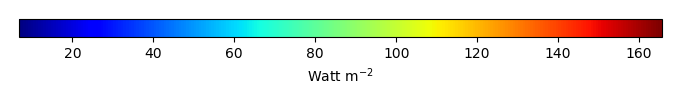MODEL MEANBIAS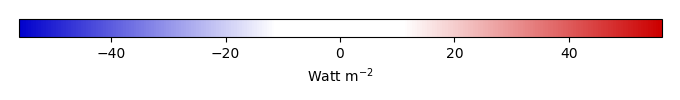BIAS SCORERMSE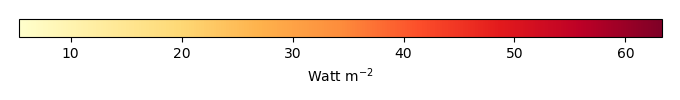RMSE SCOREBENCHMARK INTERANNUAL VARIABILITY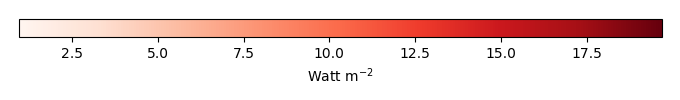MODEL INTERANNUAL VARIABILITYINTERANNUAL VARIABILITY SCOREBENCHMARK MAX MONTHMODEL MAX MONTHDIFFERENCE IN MAX MONTH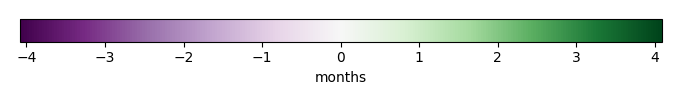SEASONAL CYCLE SCORESPATIAL TAYLOR DIAGRAMMODEL COLORS# Spatially integrated regional mean

MODEL COLORSREGIONAL MEANANNUAL CYCLEMONTHLY ANOMALYANNUAL CYCLE# All Models

BenchmarkCLM4CLM4.5CLM5# Data Information

creation_date: Mon Jun 30 23:28:16 PDT 2014

source_file: This product is generated from monthly 1 degree GEWEX SRB Radiation observations